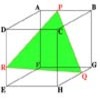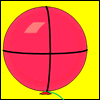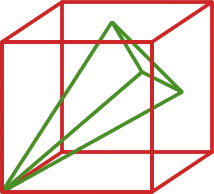#### You may also like### Cut Cube

Find the shape and symmetries of the two pieces of this cut cube.### Plane to See

P is the midpoint of an edge of a cube and Q divides another edge in the ratio 1 to 4. Find the ratio of the volumes of the two pieces of the cube cut by a plane through PQ and a vertex.### Mesh

A spherical balloon lies inside a wire frame. How much do you need to deflate it to remove it from the frame if it remains a sphere?

# Four Points on a Cube

##### Age 16 to 18 Challenge Level:

Take a cube of side length one unit. A vertex of the cube and the centres of the three faces not containing that vertex form the vertices of a tetrahedron. What is the surface area of this tetrahedron?